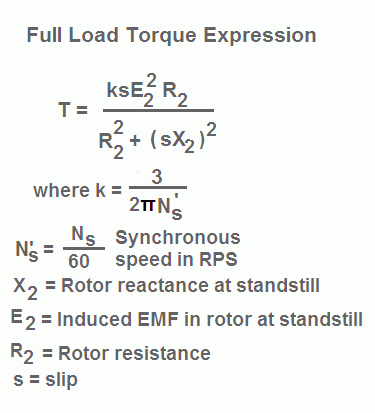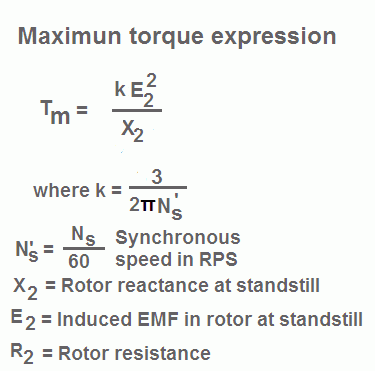# Torque Equation of Induction Motor

Full load torque equation of induction motor is as follows:# Starting Torque Equation of Induction Motor

The starting torque of a motor is that torque which is produce by it at start. It is denoted by Tst and corresponds to s = 1. So by substituting s = 1 in above expression, we get the expression for starting torque. Therefore starting torque formula for induction motor is as follows:## Maximum Torque at Start

The condition for maximum torque is

s = R2/X2  or R2 = sX2

R2 = X2 (since at start s = 1)

Thus to obtain maximum torque at the start, the value of rotor resistance must be equal to the rotor reactance at standstill.

However, the normal rotor resistance is quite small as compared to the reactance, otherwise, the rotor losses will be high and the efficiency of the motor will be low.

Hence to obtain maximum (higher) at the start some external resistance is added in the rotor circuit which is only possible in the case of slip ring induction motors. Once motor picks up speed this external resistance is reduced to zero and slip rings are short-circuited.

To obtain higher starting torque in case of squirrel cage induction motor another cage is applied on the rotor and the motor is called a double cage motor.

## Maximum Torque Formula for Induction Motor

The maximum torque is denoted by Tm and occurs at s = R2/X2. The value of slip which corresponds to maximum torque is denoted by sm. So by substituting s = R2/X2 in full load torque expression, we get the maximum torque expression. Therefore maximum torque formula for induction motor is as follows:The above expression indicates that the maximum torque Tm does not depend upon the value of rotor resistance R2.

Although Tm is independent of R2, the value of slip sm at which Tm occurs is directly proportional to the rotor resistance R2.

Moreover, the maximum torque Tm inversely proportional to the rotor reactance at standstill X2. Therefore to achieve a higher value of maximum torque, the leakage reactance of the rotor should be kept the minimum.

This is achieved by placing the rotor conductors very near to the outer periphery of the rotor and reducing the air gap between stator and rotor up to the smallest possible value.

The maximum torque Tm obtained at sm is also called pull out torque or break down torque. If the load torque increases beyond the pull-out torque then the induction motor will be pushed into the unstable region and will finally come to a standstill.

## Effect of Change in Supply Voltage on Torque

In the full load torque expression expression, as written above, E2 the rotor induced EMF is proportional E1 i.e. the stator supply voltage.

Therefore, T α  sE12

Thus torque at any speed is proportional to the square of the stator supply voltage. Therefore, when the supply voltage is changed, it changes load torque T under running condition also.

If supply voltage decreases, torque decreases abruptly and in order to maintain the same torque, slip increases or speed decreases.  Hence the motor draws extra current from the supply mains.